Model Files
water_potability_test.h5
keras
Model
deepSea Compiled Models
water_potability_test.exe
deepSea
Ubuntu

# Water Potability Test¶

Credit: AITS Cainvas CommunityPhoto by MUTI on Dribbble

# Importing Libraries¶

In :
import numpy as np
import pandas as pd
import seaborn as sns
import matplotlib.pyplot as plt
from sklearn.preprocessing import StandardScaler
from sklearn.model_selection import train_test_split
import tensorflow as tf
from tensorflow import keras
from tensorflow.keras.models import Sequential
from tensorflow.keras.layers import Dense, Dropout, Activation
from tensorflow.keras.callbacks import ModelCheckpoint


In :
!wget 'https://cainvas-static.s3.amazonaws.com/media/user_data/cainvas-admin/water_potability.csv'

--2021-08-31 18:12:16--  https://cainvas-static.s3.amazonaws.com/media/user_data/cainvas-admin/water_potability.csv
Resolving cainvas-static.s3.amazonaws.com (cainvas-static.s3.amazonaws.com)... 52.219.158.11
Connecting to cainvas-static.s3.amazonaws.com (cainvas-static.s3.amazonaws.com)|52.219.158.11|:443... connected.
HTTP request sent, awaiting response... 200 OK
Length: 525187 (513K) [application/octet-stream]
Saving to: ‘water_potability.csv.1’

water_potability.cs 100%[===================>] 512.88K  --.-KB/s    in 0.003s

2021-08-31 18:12:16 (185 MB/s) - ‘water_potability.csv.1’ saved [525187/525187]


In :
df= pd.read_csv('water_potability.csv')

In :
df

Out:
ph Hardness Solids Chloramines Sulfate Conductivity Organic_carbon Trihalomethanes Turbidity Potability
0 NaN 204.890455 20791.318981 7.300212 368.516441 564.308654 10.379783 86.990970 2.963135 0
1 3.716080 129.422921 18630.057858 6.635246 NaN 592.885359 15.180013 56.329076 4.500656 0
2 8.099124 224.236259 19909.541732 9.275884 NaN 418.606213 16.868637 66.420093 3.055934 0
3 8.316766 214.373394 22018.417441 8.059332 356.886136 363.266516 18.436524 100.341674 4.628771 0
4 9.092223 181.101509 17978.986339 6.546600 310.135738 398.410813 11.558279 31.997993 4.075075 0
... ... ... ... ... ... ... ... ... ... ...
3271 4.668102 193.681735 47580.991603 7.166639 359.948574 526.424171 13.894419 66.687695 4.435821 1
3272 7.808856 193.553212 17329.802160 8.061362 NaN 392.449580 19.903225 NaN 2.798243 1
3273 9.419510 175.762646 33155.578218 7.350233 NaN 432.044783 11.039070 69.845400 3.298875 1
3274 5.126763 230.603758 11983.869376 6.303357 NaN 402.883113 11.168946 77.488213 4.708658 1
3275 7.874671 195.102299 17404.177061 7.509306 NaN 327.459760 16.140368 78.698446 2.309149 1

3276 rows × 10 columns

In :
df.describe()

Out:
ph Hardness Solids Chloramines Sulfate Conductivity Organic_carbon Trihalomethanes Turbidity Potability
count 2785.000000 3276.000000 3276.000000 3276.000000 2495.000000 3276.000000 3276.000000 3114.000000 3276.000000 3276.000000
mean 7.080795 196.369496 22014.092526 7.122277 333.775777 426.205111 14.284970 66.396293 3.966786 0.390110
std 1.594320 32.879761 8768.570828 1.583085 41.416840 80.824064 3.308162 16.175008 0.780382 0.487849
min 0.000000 47.432000 320.942611 0.352000 129.000000 181.483754 2.200000 0.738000 1.450000 0.000000
25% 6.093092 176.850538 15666.690297 6.127421 307.699498 365.734414 12.065801 55.844536 3.439711 0.000000
50% 7.036752 196.967627 20927.833607 7.130299 333.073546 421.884968 14.218338 66.622485 3.955028 0.000000
75% 8.062066 216.667456 27332.762127 8.114887 359.950170 481.792304 16.557652 77.337473 4.500320 1.000000
max 14.000000 323.124000 61227.196008 13.127000 481.030642 753.342620 28.300000 124.000000 6.739000 1.000000
In :
df.isnull().sum()

Out:
ph                 491
Hardness             0
Solids               0
Chloramines          0
Sulfate            781
Conductivity         0
Organic_carbon       0
Trihalomethanes    162
Turbidity            0
Potability           0
dtype: int64
In :
df.Potability.value_counts().plot(kind ='pie');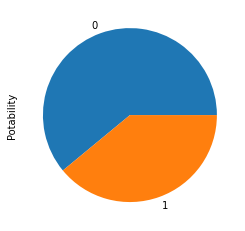# Resampling Data¶

In :
df['Potability'].value_counts()

Out:
0    1998
1    1278
Name: Potability, dtype: int64
In :
zero  = df[df['Potability']==0]   #zero values in Potability column
one = df[df['Potability']==1]  # one values in Potability column
from sklearn.utils import resample
#minority class that  is 1, we need to upsample/increase that class so that there is no bias
#n_samples = 1998 means we want 1998 sample of class 1, since there are 1998 samples of class 0
df_minority_upsampled = resample(one, replace = True, n_samples = 1998)
#concatenate
df = pd.concat([zero, df_minority_upsampled])

from sklearn.utils import shuffle
df = shuffle(df) # shuffling so that there is particular sequence

df.Potability.value_counts().plot(kind ='pie');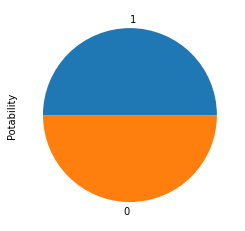# Dealing with Null Values¶

In :
from sklearn.impute import SimpleImputer
imp= SimpleImputer(strategy= 'mean')
r= imp.fit_transform(df[['ph']])
s= imp.fit_transform(df[['Sulfate']])
t= imp.fit_transform(df[['Trihalomethanes']])

In :
df['ph']=r
df['Sulfate']= s
df['Trihalomethanes']=t

In :
df.isnull().sum()

Out:
ph                 0
Hardness           0
Solids             0
Chloramines        0
Sulfate            0
Conductivity       0
Organic_carbon     0
Trihalomethanes    0
Turbidity          0
Potability         0
dtype: int64

# Correlation in Data using Heatmap¶

In :
plt.figure(figsize=(10,8))
sns.set_context('paper')
sns.heatmap(df.corr(),cmap='Blues',linecolor='White',linewidth='1',annot=True,square=True)

Out:
<AxesSubplot:>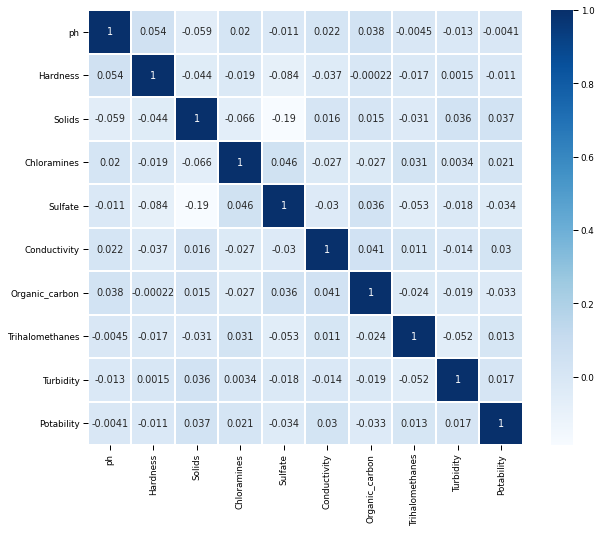Data is not correlated with each other as we can see in the Heatmap.

# Normalizing the Data¶

In :
X = df.iloc[:,:9].values
y = df.iloc[:,9:10].values

In :
#Normalizing the data
from sklearn.preprocessing import StandardScaler
sc = StandardScaler()
X = sc.fit_transform(X)
print('Normalized data:')
print(X)

Normalized data:
[ 0.45451069 -0.20395724  2.85019014  0.68753926 -1.23028959  1.67441782
0.21753713  0.29684388 -0.59419195]


# Splitting the Data¶

In :
#Train test split of model

from sklearn.model_selection import train_test_split

X_train,X_test,y_train,y_test = train_test_split(X,y,test_size = 0.1,random_state = 5)


# Building and Fitting of Model¶

In :
#importing libraries
import tensorflow as tf
from tensorflow import keras
from tensorflow.keras import layers

# creating the model

model= keras.Sequential([
layers.Dense(128, input_shape= (9,), activation= 'relu'),
layers.Dropout(0.5),
layers.Dense(64, activation= 'relu'),
layers.Dropout(0.4),
layers.Dense(32, activation= 'relu'),
layers.Dropout(0.4),
layers.Dense(1, activation= 'sigmoid')

])

model.compile(
loss= 'binary_crossentropy',
metrics= ['accuracy']
)
history= model.fit(X_train, y_train, epochs=400,validation_data=(X_test, y_test), verbose= False)
model.evaluate(X_train, y_train)

113/113 [==============================] - 0s 947us/step - loss: 0.2978 - accuracy: 0.9116

Out:
[0.29775339365005493, 0.9115684032440186]
In :
model.summary()

Model: "sequential"
_________________________________________________________________
Layer (type)                 Output Shape              Param #
=================================================================
dense (Dense)                (None, 128)               1280
_________________________________________________________________
dropout (Dropout)            (None, 128)               0
_________________________________________________________________
dense_1 (Dense)              (None, 64)                8256
_________________________________________________________________
dropout_1 (Dropout)          (None, 64)                0
_________________________________________________________________
dense_2 (Dense)              (None, 32)                2080
_________________________________________________________________
dropout_2 (Dropout)          (None, 32)                0
_________________________________________________________________
dense_3 (Dense)              (None, 1)                 33
=================================================================
Total params: 11,649
Trainable params: 11,649
Non-trainable params: 0
_________________________________________________________________

In :
model.evaluate(X_test, y_test)

13/13 [==============================] - 0s 934us/step - loss: 0.5416 - accuracy: 0.7400

Out:
[0.5416191816329956, 0.7400000095367432]

# Plotting Accuracy and Loss¶

In :
# Model Accuracy

plt.plot(history.history['accuracy'])
plt.plot(history.history['val_accuracy'])
plt.title('Model accuracy')
plt.ylabel('Accuracy')
plt.xlabel('Epoch')
plt.legend(['Train', 'Test'], loc='upper left')
plt.show()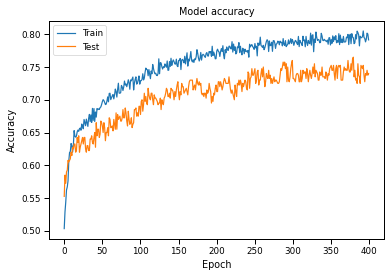In :
# Model Loss

plt.plot(history.history['loss'])
plt.plot(history.history['val_loss'])
plt.title('Model loss')
plt.ylabel('Loss')
plt.xlabel('Epoch')
plt.legend(['Train', 'Test'], loc='upper left')
plt.show()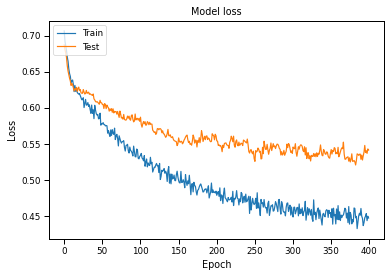# Saving the model¶

In :
model.save('water_potability_test.h5')


# Predictions¶

In :
from tensorflow.keras.models import load_model

In :
y_pred= model.predict(X_test)

In :
y_pred = (y_pred>0.5)

In :
y_pred[0:20]

Out:
array([[ True],
[ True],
[ True],
[ True],
[ True],
[False],
[ True],
[False],
[ True],
[ True],
[False],
[False],
[False],
[False],
[ True],
[ True],
[False],
[False],
[ True],
[False]])

# Deep CC¶

In :
!deepCC water_potability_test.h5

[INFO]
[SUCCESS]
Saved 'water_potability_test_deepC/water_potability_test.onnx'
[INFO]
[INFO]
Model info:
ir_vesion : 4
doc       :
[WARNING]
[ONNX]: terminal (input/output) dense_input's shape is less than 1. Changing it to 1.
[WARNING]
[ONNX]: terminal (input/output) dense_3's shape is less than 1. Changing it to 1.
WARN (GRAPH): found operator node with the same name (dense_3) as io node.
[INFO]
Running DNNC graph sanity check ...
[SUCCESS]
Passed sanity check.
[INFO]
Writing C++ file 'water_potability_test_deepC/water_potability_test.cpp'
[INFO]
deepSea model files are ready in 'water_potability_test_deepC/'
[RUNNING COMMAND]
g++ -std=c++11 -O3 -fno-rtti -fno-exceptions -I. -I/opt/tljh/user/lib/python3.7/site-packages/deepC-0.13-py3.7-linux-x86_64.egg/deepC/include -isystem /opt/tljh/user/lib/python3.7/site-packages/deepC-0.13-py3.7-linux-x86_64.egg/deepC/packages/eigen-eigen-323c052e1731 "water_potability_test_deepC/water_potability_test.cpp" -D_AITS_MAIN -o "water_potability_test_deepC/water_potability_test.exe"
[RUNNING COMMAND]
size "water_potability_test_deepC/water_potability_test.exe"
text	   data	    bss	    dec	    hex	filename
166283	   2968	    760	 170011	  2981b	water_potability_test_deepC/water_potability_test.exe
[SUCCESS]
Saved model as executable "water_potability_test_deepC/water_potability_test.exe"